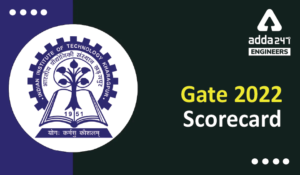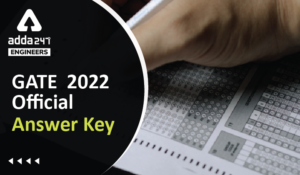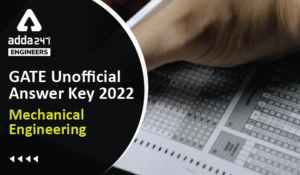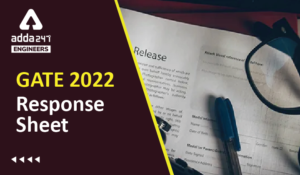Engineering Jobs   »   gate 2022, GATE 2022 ELECTRICAL, GATE...

# GATE’22 EE: Daily Practices Quiz 25-Aug-2021

GATE 2022:

GATE 2022 is being organized by IIT, Kharagpur. Through this exam, the qualified candidates get admission into master’s & direct doctoral programs in the field of Engineering/ Architecture/ Technology/ Arts & Science, etc. Qualification in GATE is mandatory for seeking admission to various institutions.

GATE’22 EE: Daily Practices Quiz 25-Aug-2021

Each question carries 2 marks
Negative marking: 0.66 mark

Total Questions: 05
Total marks: 10
Time: 12 min.

Q1. A temperature in the range of −40˚ C to 55˚ C is to be measured with a resolution of 0.1˚ C. The minimum number of ADC bits required to get a matching dynamic range of the temperature sensor
(a) 8
(b) 10
(c) 12
(d) 14

Q2. A P– type crystal is obtained by doping
1. silicon with arsenic,
2. silicon with aluminium,
3. Ge with boron,
4. Ge with phosphorus.
(a) 2 and 3 are correct
(b) 2 and 4 are correct
(c) 1 and 4 are correct
(d) 1 and 3 are correct

Q3. A 4-pole induction motor, supplied by a slightly unbalanced three-phase 50Hz source, is rotating at 1440 rpm. The electrical frequency in Hz of the induced negative sequence current in the rotor is
(a) 100
(b) 98
(c) 52
(d) 48

Q4. A network containing 50 buses in which 10 are the voltage control buses, 6 are the generator buses. Find the size of the Jacobian matrix.
(a) 33×33
(b) 34×34
(c) 83×83
(d) 84×84

Q5. A boost chopper chops 5 V DC voltage to produce an average output of 30 V. The duty ratio is:
(a) 1/6
(b) 3/6
(c) 5/6
(d) 18/3

SOLUTIONS

S1. Ans.(b)
Sol. Resolution=(analog output)/(No.of steps)
∴〖0.1〗^0 C=(55-(-40))/(2^n-1)
∴2^n-1=950
⇒n≃10 bits.

S2. Ans.(a)
Sol. Doping means the introduction of impurities into a semiconductor crystal to the defined modification of conductivity. In P-Type Semiconductor, the addition of trivalent impurities such as boron, aluminium or gallium to an intrinsic semiconductor creates deficiencies of valence electrons, called “holes”.

S3. Ans.(b)
Sol. ACQ: N_s=120f/P=(120×50)/4=1500 rpm
And N_r=1440 rpm
∴ The electrical frequency in Hz of the induced negative sequence current in the rotor
=((N_s+N_r)/N_s )×50=((1500+1440)/1500)×50=98 Hz

S4. Ans.(c)
Sol. Size of Jacobian matrix=(2n-m-2)×(2n-m-2)
Where, n=number of buses and m=number of PV buses=10+6-1=15
∴ Size of Jacobian matrix=(2n-m-2)×(2n-m-2)
=(2*50-15-2) × (2*50-15-2)
=83×83

S5. Ans.(c)
Sol. Duty ratio(D)=1-V_in/V_out =1-5/30=5/6

Sharing is caring!

Thank You, Your details have been submitted we will get back to you.
•HURL Recruitment 2022 Apply Online for 1...
•GATE 2022 Scorecard Released, Check Step...
•GATE Result 2022 OUT Live Check Your GAT...
•GATE 2022 Official Answer Key Out, Downl...
•GATE 2022 Answer Key Mechanical Engineer...
•GATE 2022 Response Sheet, Direct Link to...